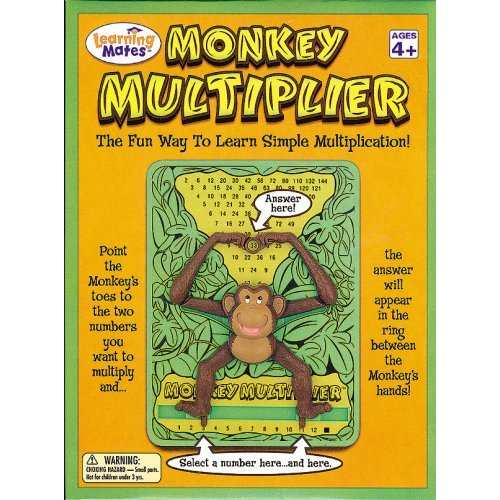The Fun Way To Learn Simple Multiplication!!!!!
Ages: 4 onwards

Learning one’s multiplication tables for numbers one through twelve can be a tedious task for many children. With this in mind, ‘Learning Mate‘ have decided to put the fun back into math! Monkey Multiplier uses a cute little monkey to help your child develop both an interest and confidence in mathematics.

Just point the monkey’s toes to the two numbers you want to multiply and the answer will mysteriously appear in the ring between his hands. The mechanical movement of the monkey’s hands provides the correct answer.

This product is a great way to introduce the idea of a calculator. It is actually more fun than an electronic calculator because the child can see the relation between the two numbers that are multiplied. The product of the two numbers can be seen in a systematic and visual manner when the hands of the monkey move towards the correct answer. Children will have fun learning their multiplication tables and checking their homework.

Suggested ways to use Monkey Multiplier:

• List down multiplication tables beginning with the table of 1. e.g. 1X1 = 1×2 etc